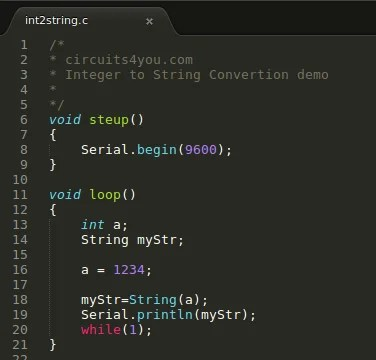# How to convert integer to string and string to int on Arduino ?

New version of Arduino supports String data type . In this tutorial we will see both integer to string and string to integer conversion. Conversion of integer to string can be done using single line statement.

Example 1: Integer to String Conversion Arduino
int a = 1234;
String myStr;
myStr = String(a);   //Converts integer to string

Example 2: String to Integer conversion Arduino
String val = “1234”;
int result = val.toInt();  //Converts string to integer

##Overview of Strings in C

In the Arduino C language, a string is the type used to store any text including alphanumeric and special characters. Internally, it’s represented as an array of characters. Each string is terminated by a ‘null’ character. They are called “null-terminated strings.” Each character is enclosed within single quotes whereas a string is enclosed with double quotes. Many Arduino C programs make use of strings and associated properties. The operations possible on strings include- calculating the string length, concatenation of strings, comparison of strings and so on.

### What is Type Conversion?

Many times in C programs, expressions contain variables and constants of different data types. For calculation purposes, they need to be converted to the same data type. When you convert one data type into another, the method is termed type conversion.

In Arduino C, we have 2 types of type conversion

Implicit Type Conversion – This kind of type conversion is done automatically by the compiler. Programmers do not play any role here.

Explicit Type Conversion– Here the programmer is responsible for the type conversion. This is also called typecasting. The syntax is as follows.

(datatype) expression;

The above item is called a cast operator. Take a look at this example.

char a;
int b;
a=(char)b;

This is a simple way to convert an integer to a character type. Here, ‘a’ is of character data type and b is of integer data type. It is not possible to assign the value of variable b to variable a as they are of different data types. So, we typecast integer b to character in this example. Now, both a and b are of character data type.

### How to Convert String to Integer in the Arduino ?

Sometimes, a number is input as a string. To use it for any mathematical operation, we have to convert the string to integer. There are two ways to do this.

The first method is to manually convert the string into an integer.
The second method is to use the built-in functions.

Manual Conversion

Below is a list of ASCII (American Standard Code for Information Interchange) characters and their decimal value.

ASCII Character Decimal Value

0 = 48
1 = 49
2 = 50
3 = 51
4 = 52
5 = 53
6 = 54
7 = 55
8 = 56
9 = 57

Numbers are stored in character format within the string. In order to get the decimal value of each string element, we have to subtract it with decimal value of character ‘0.’ Let’s make this clear with the help of an example.

Example 1: Program to Manually Convert a String to an Integer

```/*
* circuits4you.com
* String to Integer Manual Conversion
*/

void setup()
{
Serial.begin(9600);
}

void loop()
{
String num = "1234";
int i, len;

int result=0;

Serial.print("Number: ");
Serial.println(num);

len = num.length();

for(i=0; i<len; i++)
{
result = result * 10 + ( num[i] - '0' );
}

Serial.println(result);
}```

The program code is written within the curly braces of the main function. Inside the loop function we first define and declare the different variables along with their data types. Variables i, len and result are declared as of integer data type. The result variable is initialized to zero. The serial.print() function is then called to display the message “number” on the terminal screen.  In this case, the string is an array of characters pointed to by num. Then, we calculate the length of the string using the length() function. Next, we loop through the string and convert the string into decimal value. Finally, the string is converted into an integer and printed on the screen.

Example 2: A Program to Convert String to Integer Using the atoi() Function

atoi() is a function that converts a string data type to integer data type in the C language. The syntax of this function is as follows

int atoi((const char * str);

Here, str is of type pointer to a character. The const keyword is used to make variables non-modifiable. This function returns an integer value after execution. The atoi() function is present in the stdlib.h header file. This header file contains all the type casting functions used in the C language.

```void setup()
{
Serial.begin(9600);
}

void loop()
{
char x = "450";
int result = atoi(x);
Serial.print("integer value of the string is: ");
Serial.println(result);
while(1);
}```

The list of other string to numerical values in-built type casting functions used in C programs include

atof()– This function is used to convert string to a floating point value.
atol()– Use this function to convert a string to a long integer value.

Hope this article was helpful and informative. Do try out the examples for yourself and experiment with them. Programming is one of those things that becomes better with practice.